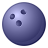# Linear Referencing and calculating new polyline values

1669
5
11-09-2016 01:36 PMNew Contributor III
Please see attached.  I was able to Aggregate Polyline segments and then split the polyline into Urban and Rural sections, but when I split the lines between rural and urban areas, the fields that came up were for the Cities boundary layer or ? and not for the Polyline layer (postmiles).
What I need to do is re-calculate (Calibrate?) the routes based on the Postmile measures in the new Urban and Rural Segments so that the Begin/End measures are from Boundary INTERSECTION POINT To BOUNDARY INTERSECTION POINT?
Locate Features along Routes is manual and not efficient for what I need help with.
If I can aggregate segments and then break them up into Urban and Rural Segments, I am 2/3 of the way there.
What functionality in Linear Referencing can I use?
Thanks,

Daniel T. Brenner

RESEARCH ANALYST I (GEOGRAPHIC INFORMATION SYSTEMS)

DIVISION OF RESEARCH, INNOVATION, and SYSTEM INFORMATION

Highway System Information and Performance

1120 N Street MS 38

Sacramento, CA  95814

(916) 657-4277

(510) 593-7547 cell

daniel.brenner@dot.ca.gov

“Consider the unconsidered.”

“Certainty is not a sure thing!”

5 RepliesbyMVP Honored Contributor

Are the lines that you split your M-Aware Routes?  If they are, then the split lines contain the measures at each line end that you are looking for.  In that case you just need to do a Python calculation on each line to get the From field using !Shape.FirstPoint.M! and to get the To field using !Shape.LastPoint.M!.New Contributor III

I'm not sure what you mean by M aware routes but for now maybe you can

For example:

I have 2 segments. The first one measures are 0 to 4, the second one is 4

to 8. The point of where the line intersects the polygon is at 6.

If the line is aggregated ( where the wouldn't be two segments and the

values would be 0 to x or in this case 8), would the intersecting point

still be 6 or would it be...?

The other question I have would be how to show the point of intersection as

having the From and To fields with values of From = 0 and To = 6 and From =

6 and To = 8?

I'm out today and Wednesday but will get back to you soon.byMVP Honored Contributor

Have you ever used the Create Routes tool to aggregate your segments?  This tool not only joins segments together, it adds measure (M) coordinate values (M is store the same way that X, Y or Z coordinates are stored) to create M-Aware polylines based on several methods (frequently using line length).  If you don't have a set of M-Aware Route polylines you cannot use Linear Referencing tools like Locate Features along Routes.

It is unclear what you mean by Aggregating lines.  Aggregating lines using tools like Dissolve that do not add M coordinates to polylines is not linear referencing.  Nor is just by assigning many lines a common value, but leaving them as separate lines.

Each coordinate of the polyline is assigned a measure or M value.  In the simplest case, if you had a two point line with a starting coordinate with a measure of 0 and and ending coordinate with a measure of 8, if you split that line, the coordinate created at the split will interpolate the distance of that point between 0 and 8.  So if the split was at measure position 6, the line with a starting measure of 0 would now end at 6, and the line with an ending measure of 8 would now start at 6.

I gave you the Python calculation to get these coordinates into a From and To field already, if your lines are M-Aware Routes.  Read more on Linear Referencing if you still do not understand what an M-Aware Route is, since they are the foundation of LR.New Contributor III

Richard,

Thanks for all of this. I will work with it when I am in the office on

Thursdsy, but I will say that I did use the Make Routes tool in LR but I

didn't get any Measure values for some reason (just ID fields)???

If there is a better term other than Aggregation for adding measure values

for a poly line with multiple segments together into one measure, please

enlighten me??

Will resoon better on Thursday.

Thank you for your time and effort.

Daniel BrennerbyMVP Honored Contributor

Create Routes does not create fields with measures in them, nor does any other tool.  Create Routes creates coordinates with measures stored in the geometry of the line, just like the X and Y coordinates at every point that makes up the line.

It is your job to create the From_M and To_M fields and calculate the M coordinates into them.  You would do the same thing if you wanted to have fields display the X and Y coordinates for the start and end point of the line (although, unlike the M coordinates, for X and Y coordinates there may be other tools that also can accomplish this task).  The fields you would create to store all of the coordinate types would all be double fields.  Below are sample coordinate fields for all the possible coordinate values of the points at the start and end of your polylines that you could create and the Python calculations you would need to run to populate them.

Polyline From coordinate calculations:

FROM_X field is calculated using a Python calculation of !Shape.FirstPoint.X!

FROM_Y field is calculated using a Python calculation of !Shape.FirstPoint.Y!

FROM_Z field is calculated using a Python calculation of !Shape.FirstPoint.Z!

FROM_M field is calculated using a Python calculation of !Shape.FirstPoint.M!

Polyline To coordinate calculations:

TO_X field is calculated using a Python calculation of !Shape.LastPoint.X!

TO_Y field is calculated using a Python calculation of !Shape.LastPoint.Y!

TO_Z field is calculated using a Python calculation of !Shape.LastPoint.Z!

TO_M field is calculated using a Python calculation of !Shape.LastPoint.M!